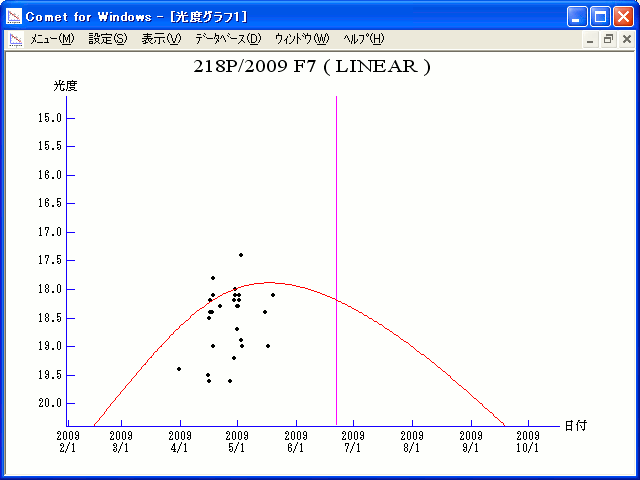# \$B%j%K%"WB@1(B

218P/LINEAR (2009)###\$B%W%m%U%#!<%k(B

 \$BId9f(B 218P/2009 F7 \$B8!=PF|(B 2009\$BG/(B3\$B7n(B31\$BF|!"(B4\$B7n(B15\$BF|(B \$B8!=P8wEY(B 19.4\$BEy!"(B19.5\$BEy(B \$B8!=P LINEAR, G. Sostero, E. Prosperi, E. Guido, P. Camilleri (Grove Creek Observatory, Trunkey, New South Wales)

###\$B###\$B50F;MWAG(B

```                    Epoch = 2009 June 18.0 TT
T = 2009 June 22.28618 TT        Peri. =  10.60506
e = 0.4902703                    Node  = 226.74365 2000.0
q = 1.7014372 AU                 Incl. =  18.15146
a =  3.3379202 AU   n = 0.16161815   P =   6.10 years
```

###\$B8wEYJQ2=(B

```        m1 = 13.5 + 5 log\$B&\$(B + 20.0 log r
```##### \$B50F;MWAG\$O!"(BCBET 1767\$B\$K7G:\\$5\$l\$?\$b\$N\$G\$9!#(B \$B8wEY%0%i%U\$O(BComet for Windows\$B\$G:n@.\$7\$?\$b\$N\$G\$9!#(B